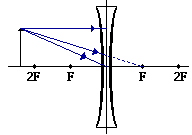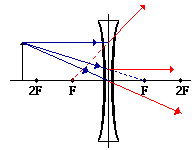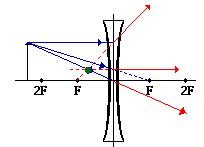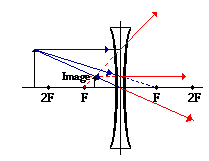Rules for ray tracing a converge lens

• Any incident ray traveling parallel to the principal axis of a diverging lens will refract through the lens and travel in line with the focal point (i.e., in a direction such that its extension will pass through the focal point).
• Any incident ray traveling towards the focal point on the way to the lens will refract through the lens and travel parallel to the principal axis.
• An incident ray that passes through the center of the lens will in affect continue in the same direction that it had when it entered the lens.
•  1. Pick a point on the top of the object and draw three incident rays traveling towards the lens.Using a straight edge, accurately draw one ray so that it travels towards the focal point on the opposite side of the lens; this ray will strike the lens before reaching the focal point; stop the ray at the point of incidence with the lens. Draw the second ray such that it travels exactly parallel to the principal axis. Draw the third ray to the exact center of the lens. Place arrowheads upon the rays to indicate their direction of travel.

2. Once these incident rays strike the lens, refract them according to Snell's law.

The ray that travels towards the focal point will refract through the lens andtravel parallel to the principal axis. Use a straight edge to accurately draw its path. The ray that traveled parallel to the principal axis on the way to the lens will refract and travel in a direction such that its extension passes through the focal point on the object's side of the lens. Align a straight edge with the point of incidence and the focal point, and draw the second refracted ray. The ray that traveled to the exact center of the lens will continue to travel in the same direction. Place arrowheads upon the rays to indicate their direction of travel. The three rays should be diverging upon refraction.

3. Locate and mark the image of the top of the object.

The image point of the top of the object is the point where the three refracted rays intersect.Since the three refracted rays are diverging, they must be extended behind the lens in order to intersect. Using a straight edge, extend each of the rays using dashed lines. Draw the extensions until they intersect. All three extensions should intersect at the same location. The point of intersection is the image point of the top of the object. The three refracted rays would appear to diverge from this point. This is merely the point where all light from the top of the object would appear to diverge from after refracting through the double concave lens. Of course, the rest of the object has an image as well and it can be found by applying the same three steps to another chosen point.

4. Repeat the process for the bottom of the object.

The goal of a ray diagram is to determine the location, size, orientation, and type of imagethat is formed by the double concave lens. Typically, this requires determining where the image of the upper and lower extreme of the object is located and then tracing the entire image. After completing the first three steps, only the image location of the top extreme of the object has been found. Thus, the process must be repeated for the point on the bottom of the object. If the bottom of the object lies upon the principal axis (as it does in this example), then the image of this point will also lie upon the principal axis and be the same distance from the lens as the image of the top of the object. At this point the complete image can be filled in.

Rules for ray tracing a diverging lens Provided By Physics Classroom

The focal point is located 20.0 cm from a double concave lens. An object is placed 12 cm from the lens. Determine the image distance.

Given: f = -20.0 cm and do = +12.0 cm

(Careful: double concave lens have focal lengths which are negative.)

Use the equation 1 / f = 1 / do + 1 / di

Substitute and solve for di.

1 / (-20.0) = 1 / (12.0) + 1 / di

1 / (-20.0) - 1 / (12.0) = 1 / di

-0.1333 cm-1 = 1 / di

di = 1 / (-0.1233 cm-1)

di = -7.5 cm

Example Provide by Physics Classroom